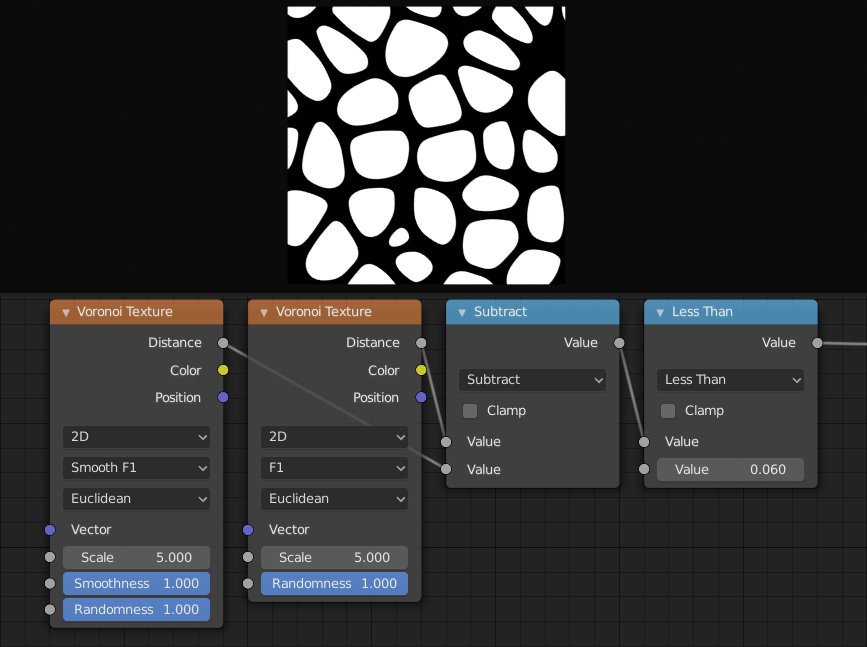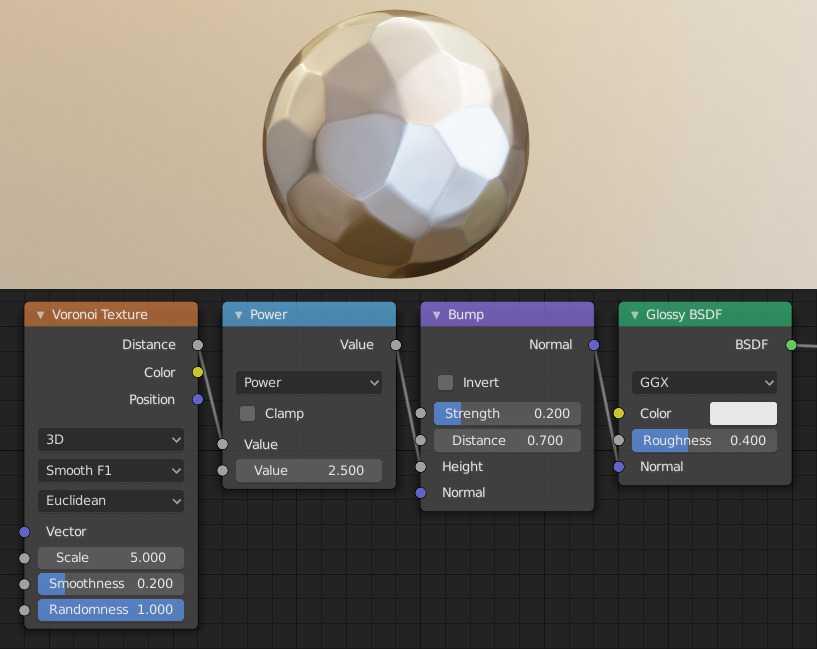# Voronoi Texture Node¶Voronoi Texture Node.

The Voronoi Texture node evaluates a Worley Noise at the input texture coordinates.

## Inputs¶

The inputs are dynamic, they become available if needed depending on the node properties.

Vector

Texture coordinate to evaluate the noise at; defaults to Generated texture coordinates if the socket is left unconnected.

W

Texture coordinate to evaluate the noise at.

Scale

Scale of the noise.

Smoothness

The smoothness of the noise.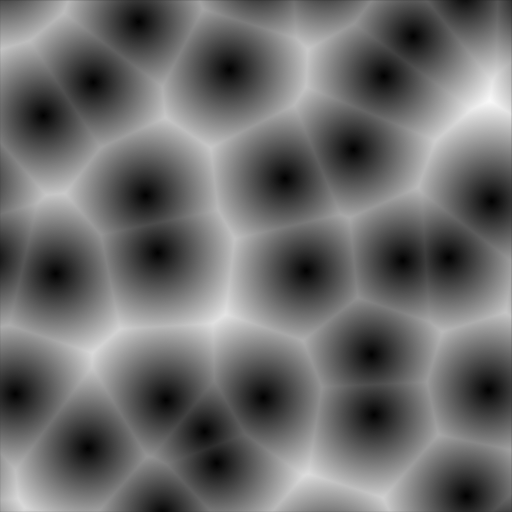Smoothness: 0.0.¶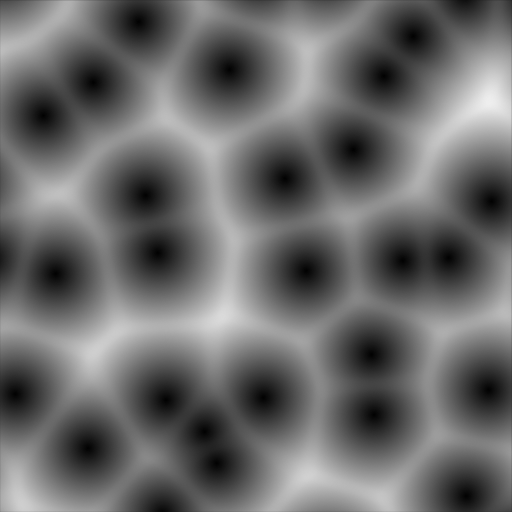Smoothness: 0.25.¶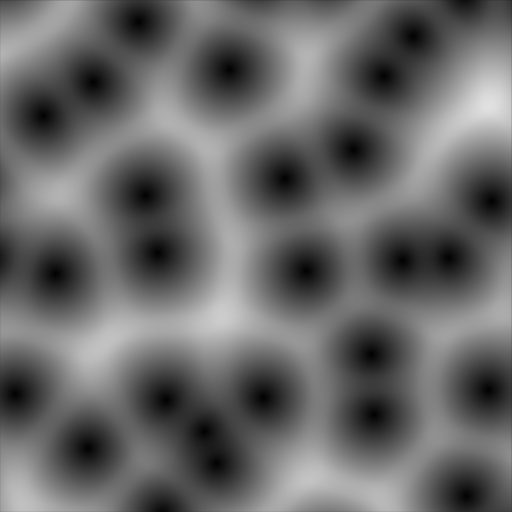Smoothness: 0.5.¶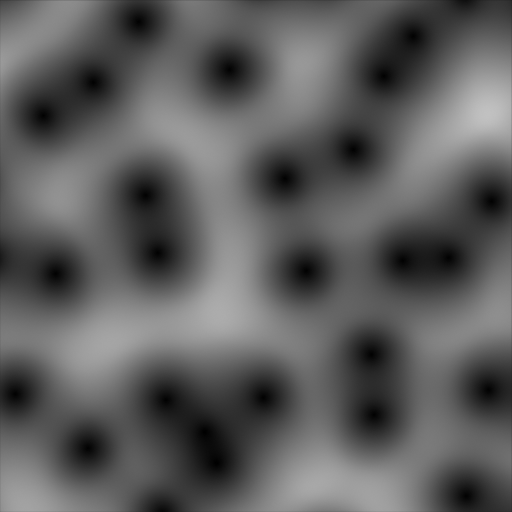Smoothness: 1.0.¶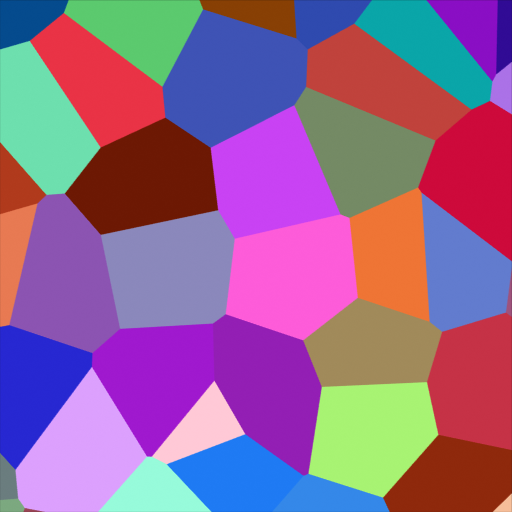Smoothness: 0.0.¶Smoothness: 0.25.¶Smoothness: 0.5.¶Smoothness: 1.0.¶
Exponent

Exponent of the Minkowski distance metric.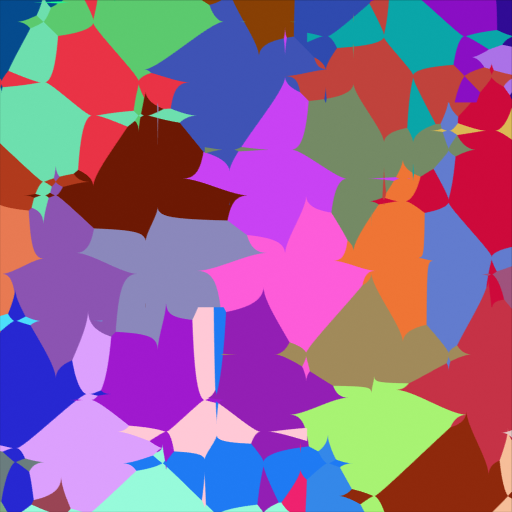Exponent: 0.5.¶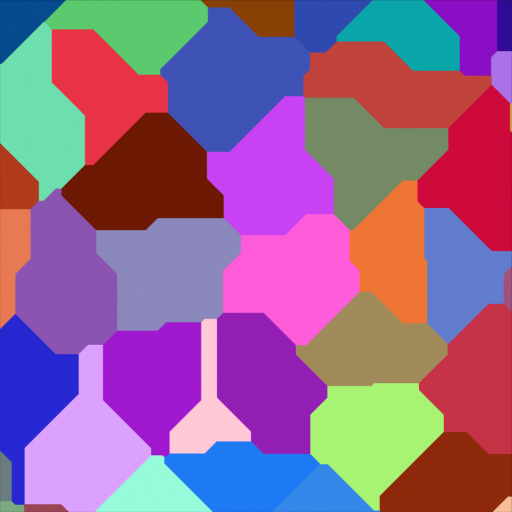Exponent: 1.0.¶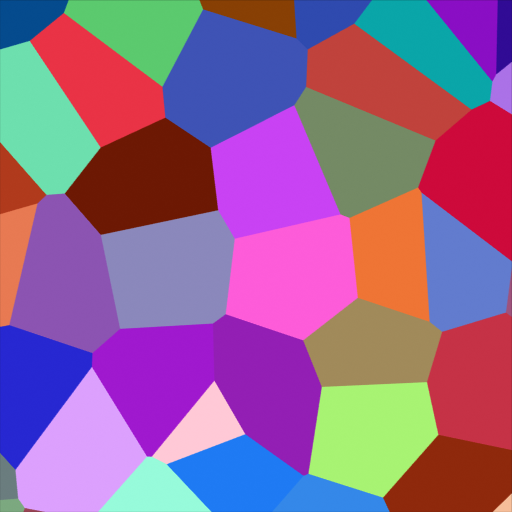Exponent: 2.0.¶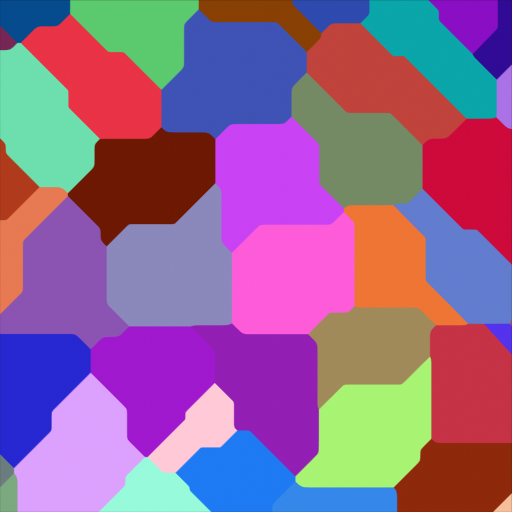Exponent: 32.0.¶
Randomness

The randomness of the noise.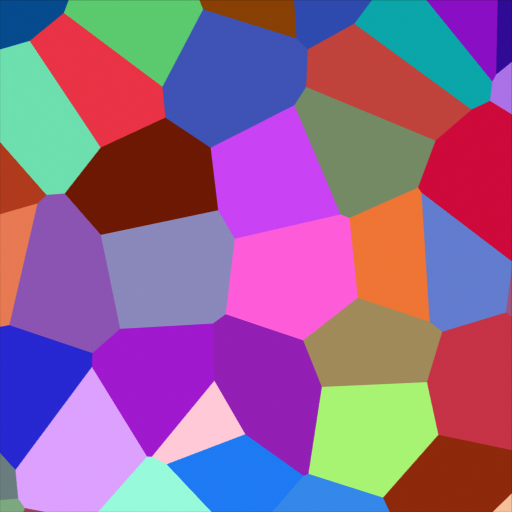Randomness: 1.0.¶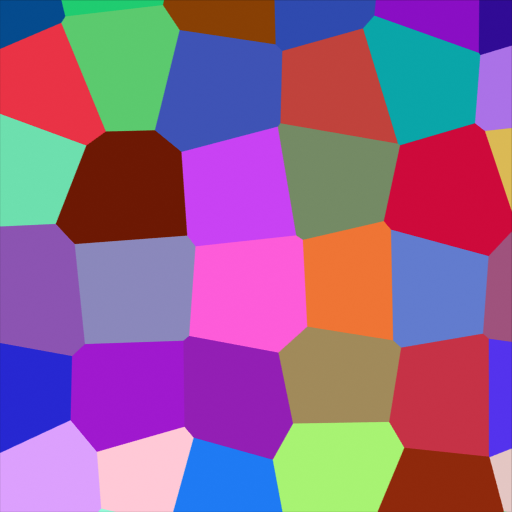Randomness: 0.5.¶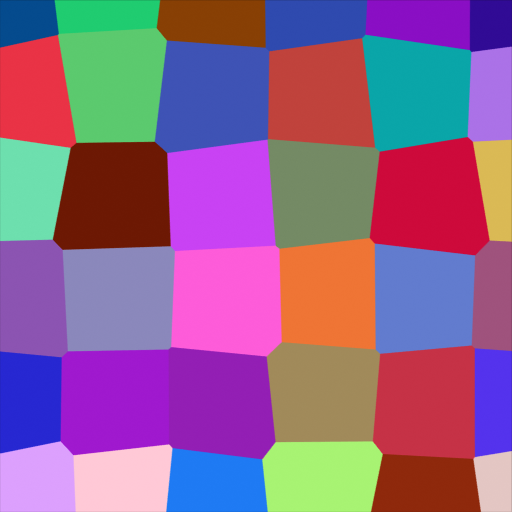Randomness: 0.25.¶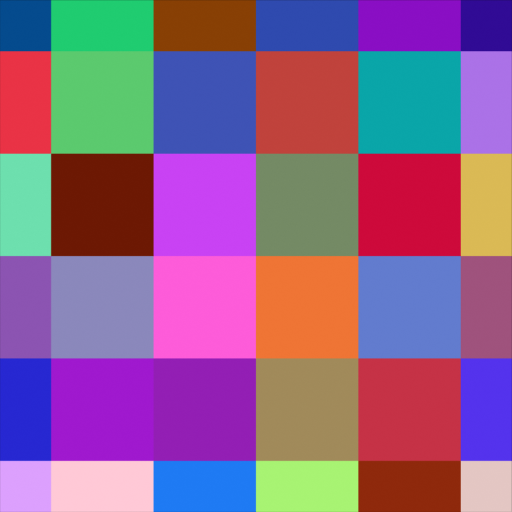Randomness: 0.0.¶

## Properties¶

Dimensions

The dimensions of the space to evaluate the noise in.

1D

Evaluate the noise in 1D space at the input W.

2D

Evaluate the noise in 2D space at the input Vector. The Z component is ignored.

3D

Evaluate the noise in 3D space at the input Vector.

4D

Evaluate the noise in 4D space at the input Vector and the input W as the fourth dimension.

Higher dimensions corresponds to higher render time, so lower dimensions should be used unless higher dimensions are necessary.

Feature

The Voronoi feature that the node will compute.

F1

The distance to the closest feature point as well as its position and color.Distance.¶Color.¶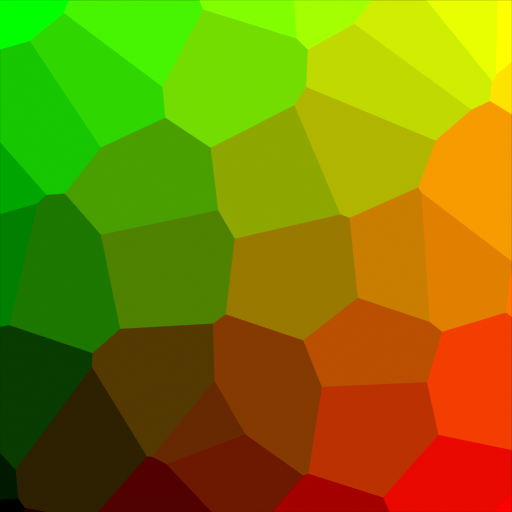Position.¶
F2

The distance to the second closest feature point as well as its position and color.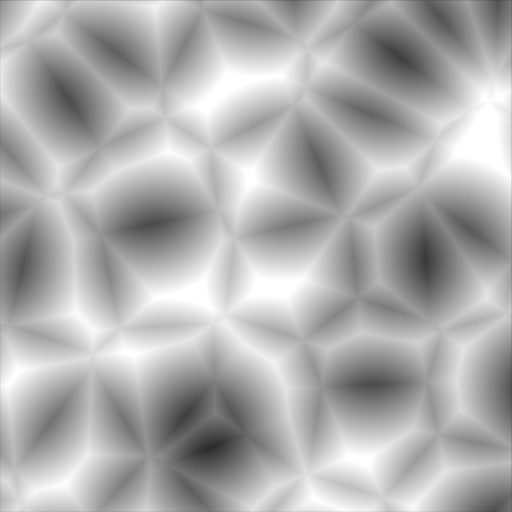Distance.¶Color.¶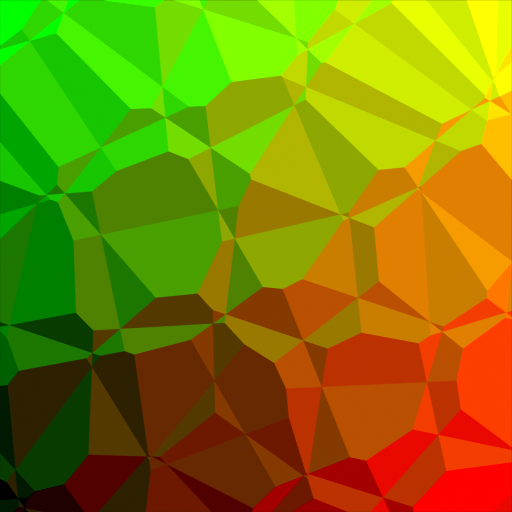Position.¶
Smooth F1

A smooth version of F1.Distance.¶Color.¶Position.¶
Distance to Edge

The distance to the edges of the Voronoi cells.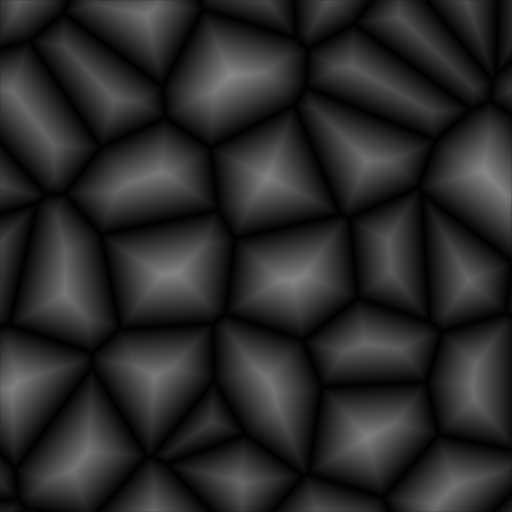Distance.¶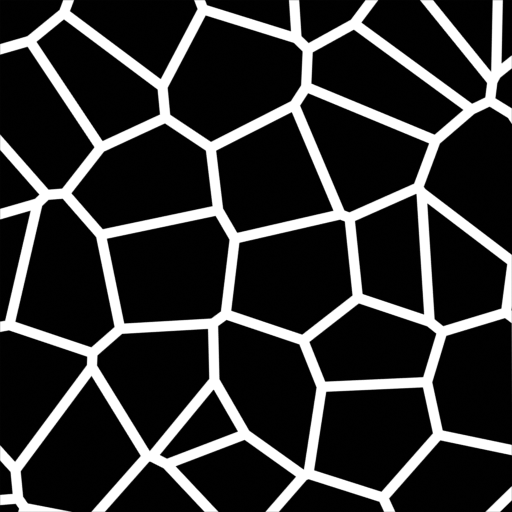Distance smaller than 0.05.¶

The radius of the n-sphere inscribed in the Voronoi cells. In other words, it is half the distance between the closest feature point and the feature point closest to it.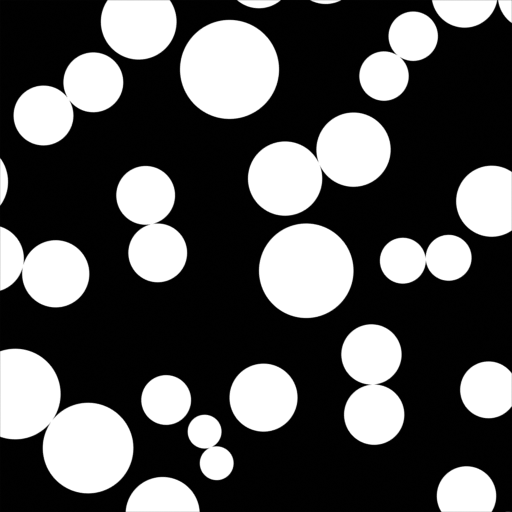The n-sphere radius can be used to create tightly packed n-spheres.¶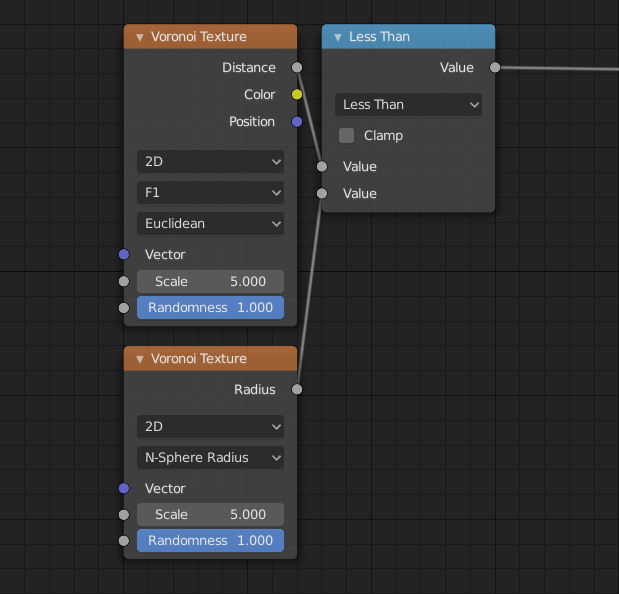Node tree for the shader to the left.¶
Distance Metric

The distance metric used to compute the texture.

Euclidean

Use the Euclidean distance metric.

Manhattan

Use the Manhattan distance metric.

Chebychev

Use the Chebychev distance metric.

Minkowski

Use the Minkowski distance metric. The Minkowski distance is a generalization of the aforementioned metrics with an Exponent as a parameter. Minkowski with an exponent of one is equivalent to the Manhattan distance metric. Minkowski with an exponent of two is equivalent to the Euclidean distance metric. Minkowski with an infinite exponent is equivalent to the Chebychev distance metric.Minkowski Exponent: 0.5 (Minkowski 1/2).¶Minkowski Exponent: 1.0 (Manhattan).¶Minkowski Exponent: 2.0 (Euclidean).¶Minkowski Exponent: 32.0 (approximation of Chebychev).¶

## Outputs¶

Distance

Distance.

Color

Cell color. The color is arbitrary.

Position

Position of feature point.

W

Position of feature point.